Win up to 100% scholarship on Aakash BYJU'S JEE/NEET courses with ABNAT Win up to 100% scholarship on Aakash BYJU'S JEE/NEET courses with ABNAT

# Chemical Equilibrium Formulas For NEET

When the system consists of more than one substance and the composition of the system does not vary with time, the system is said to be in chemical equilibrium. The chemical composition of a system at equilibrium must be uniform and there should be no net chemical reaction taking place.

## Types of Reaction

1. Reversible reaction
2. Irreversible reaction

## State of Equilibrium

Most of the chemical reactions do not go to completion in a closed system and attain a state of equilibrium.

Another approach comes from kinetics as developed by Guldberg and Waage (1863). Equilibrium is said to have reached in a physical or chemical system when the rate of forward and reverse processes are equal.

At equilibrium,

Rate of forward reaction = Rate of backward reaction

## Law of Chemical Equilibrium or Mass Action

At equilibrium,

rf = rb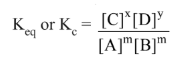Where Kc = kf / kb

Where [ ] is used to indicate “molar concentration”.

## Equilibrium Constant in terms of Partial Pressure

In a gaseous system, we can use partial pressure terms in places of active masses. Thus, for the above gaseous equilibrium,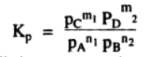Where Kp is an equilibrium constant in terms of partial pressure.

## Equilibrium Constant in terms of Mole Fraction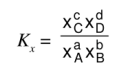Where Kx is the equilibrium constant in terms of mole fraction.

## Relation between Kp and Kc

Kp = Kc (RT)Δn

where R is gas constant 0.0821 liter.atm/degree/mole and Δn is the total number of molecules of the product – total number of molecules of the reactants.

## Relation between Equilibrium Constant and Standard Free Energy

ΔGo = – RT ln Keq

(or)

ΔGo = – 2.303RT log Keq

## Reaction Quotient (Q)

The reaction quotient has the same algebraic form as Keq but the current concentrations nor specifically the equilibrium concentrations are used in calculations.

The expression for Q is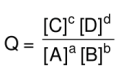## Degree of Dissociation

Degree of dissociation (ɑ) = No. of molecules dissociated/Total no.of molecules taken.

Dissociation constant, Kdiss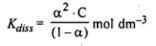## Factors affecting Equilibrium

### Le Chatelier’s Principle

If an equilibrium system is subjected to a change of any one of the factors such as temperature, pressure or concentration the equilibrium shifts in the direction that ends the annual effect of that change.

Important conclusions of the principle are

### Pressure change

The increase of pressure of an equilibrium system shifts the equilibrium in the direction in which there is a decrease in volume.

### Temperature change

The increase of temperature favours the reaction which takes place with the absorption of heat. The effect of a decrease in temperature is just the reverse of it.

### Concentration

When a substance is added its active mass increases. Hence the reaction will proceed in the direction in which the increase in active mass is used up.

### Effect of adding an inert gas

At constant volume equilibrium is unaffected. At constant pressure, the volume of the system increases.

## Thermodynamics of Equilibrium

Van’t Hoff Equation =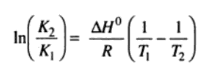(or)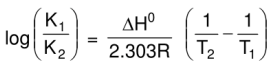Chemical equilibrium is the stage of a reversible reaction at which the active masses of the reactants and the products become constant in the mixture and do not change with time. It is the state of a reversible reaction at which measurable properties like colour, density, pressure and concentration are nearly unchangeable.

### Recommended Video:

How to Master Physical Chemistry for NEET | NEET 2023 Chemistry Preparation | Aakash Byju’s NEET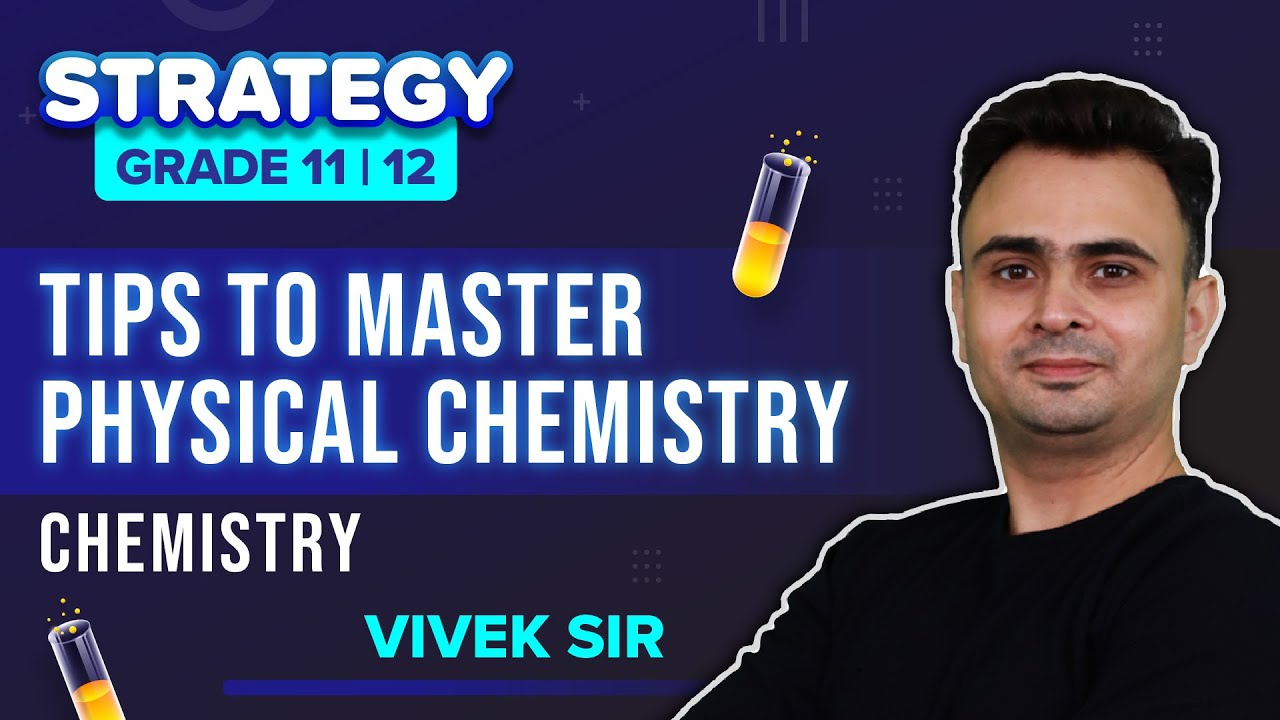Suggested NEET Chemistry articles: